# Limits and Continuity Questions and Answers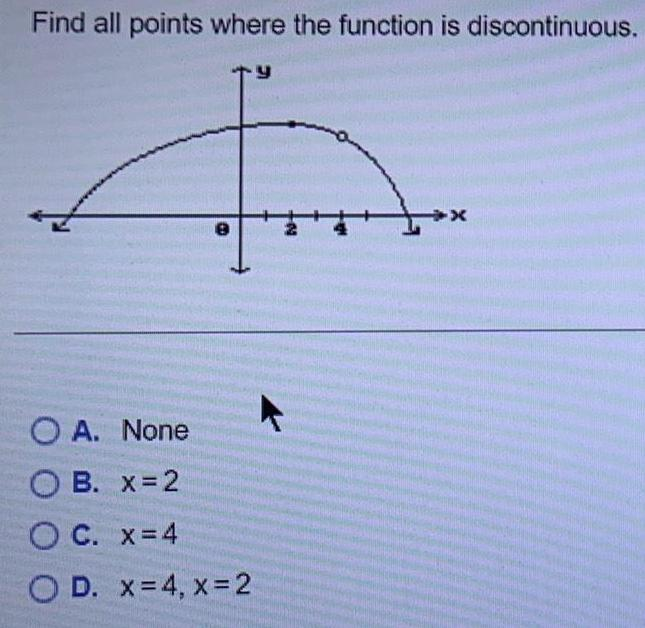Math
Limits and Continuity
Find all points where the function is discontinuous. A. None B. x=2 C. x = 4 D. x = 4, x = 2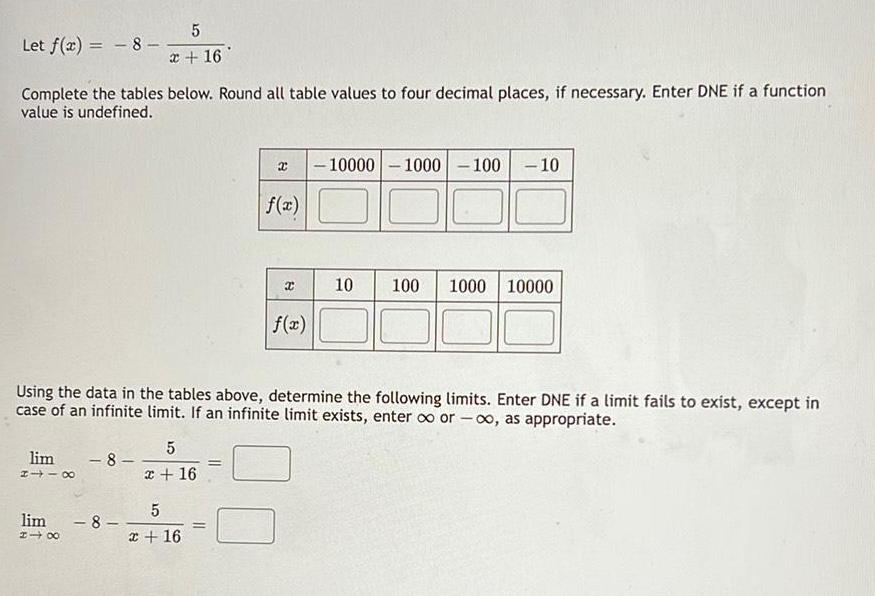Math
Limits and Continuity
Let f(x) = -8 - 5/x+16 Complete the tables below. Round all table values to four decimal places, if necessary. Enter DNE if a function value is undefined. Using the data in the tables above, determine the following limits. Enter DNE if a limit fails to exist, except in case of an infinite limit. If an infinite limit exists, enter ∞ or -∞, as appropriate.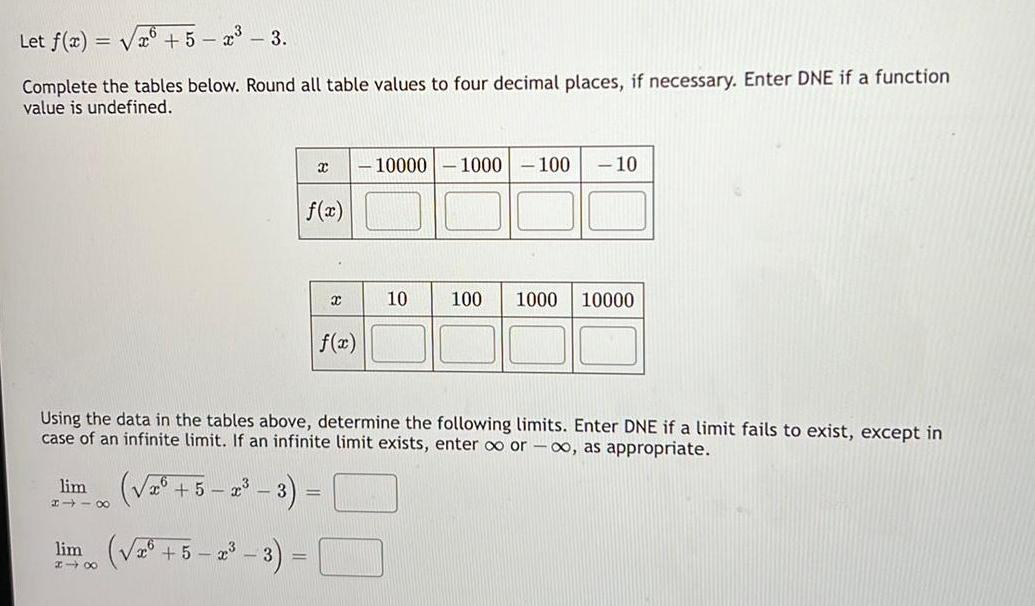Math
Limits and Continuity
Let f(x)=√x6 +5x - x³ - 3. Complete the tables below. Round all table values to four decimal places, if necessary. Enter DNE if a function value is undefined. Using the data in the tables above, determine the following limits. Enter DNE if a limit fails to exist, except in case of an infinite limit. If an infinite limit exists, enter ∞ or -∞, as appropriate.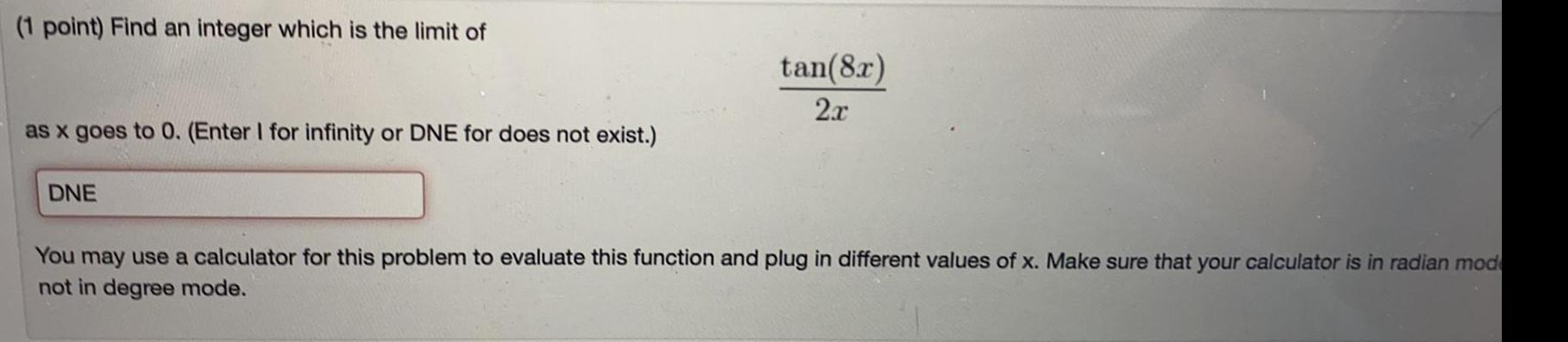Math
Limits and Continuity
(1 point) Find an integer which is the limit of tan(8x)/2x as x goes to 0. (Enter I for infinity or DNE for does not exist.)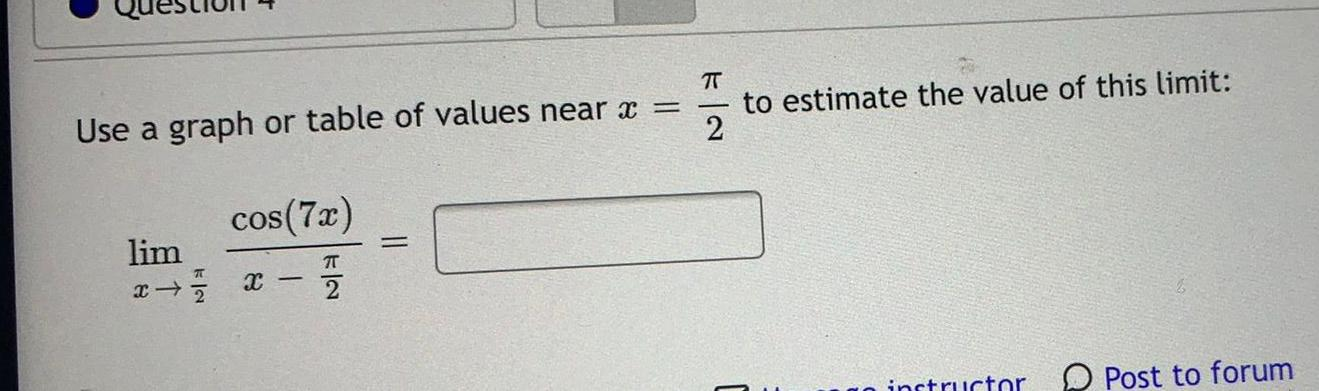Math
Limits and Continuity
Use a graph or table of values near x =π/2 to estimate the value of this limit: lim x - 72 cos(7x)/x-π/2 i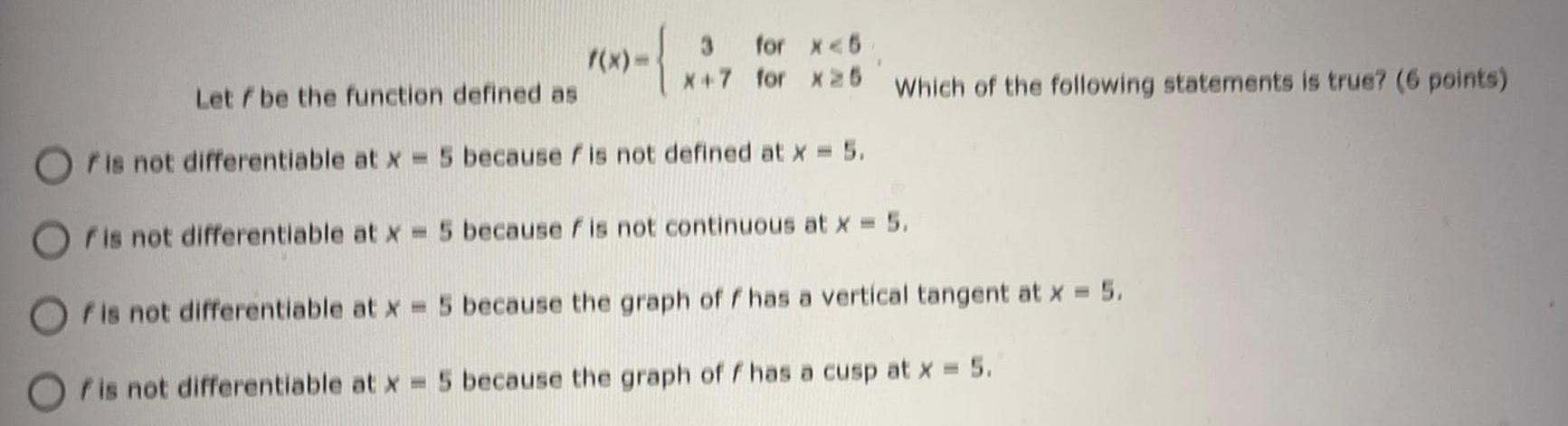Math
Limits and Continuity
Let f be the function defined as f(x)= 3 for x<5 x+7 for x≥ 5 Which of the following statements is true? f is not differentiable at x = 5 because f is not defined at x = 5. f is not differentiable at x = 5 because fis not continuous at x = 5. f is not differentiable at x = 5 because the graph off has a vertical tangent at x = 5. f is not differentiable at x = 5 because the graph of f has a cusp at x = 5.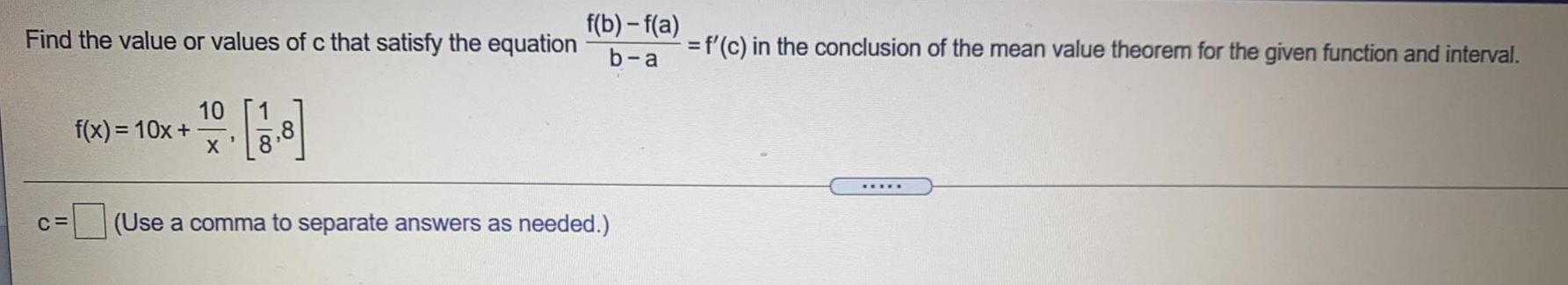Math
Limits and Continuity
Find the value or values of c that satisfy the equation f(b)-f(a) / b-a = f'(c) in the conclusion of the mean value theorem for the given function and interval. f(x)=10x+10/x , [1/8,8] c= (Use a comma to separate answers as needed.)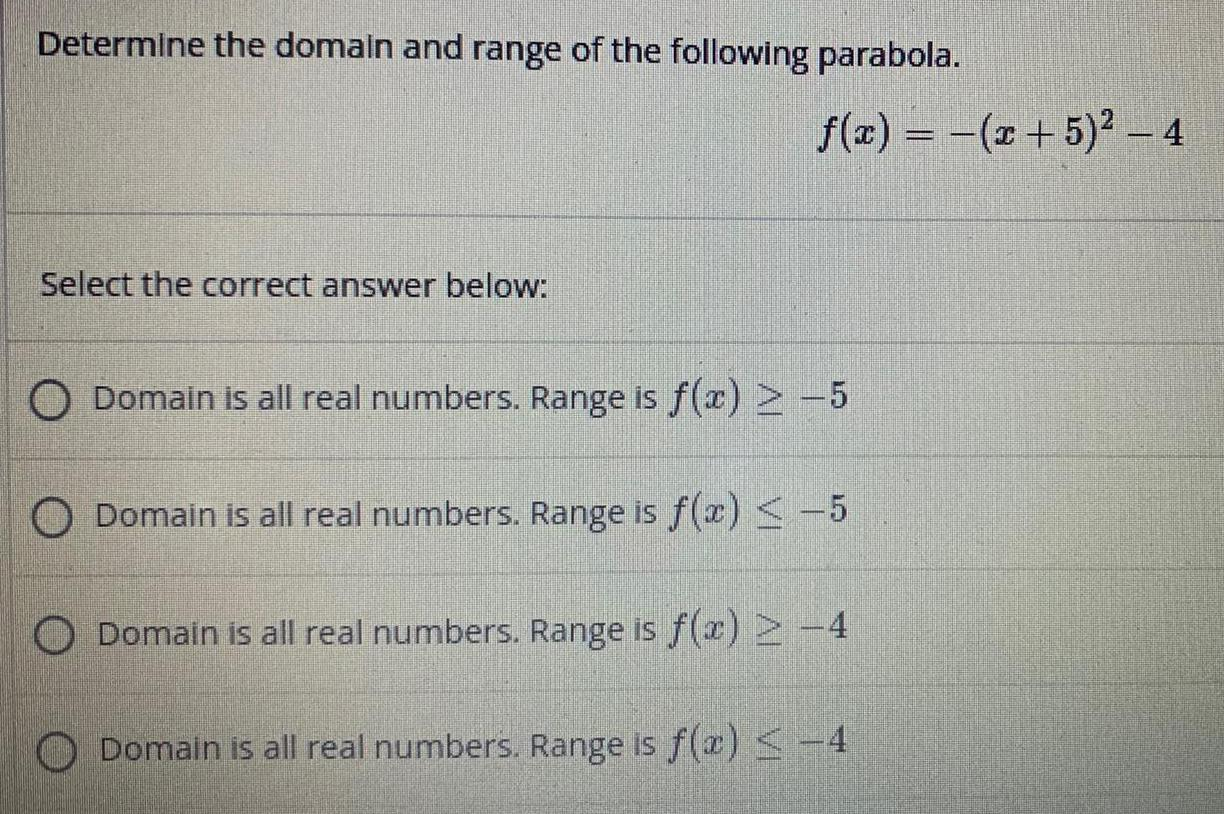Math
Limits and Continuity
Determine the domain and range of the following parabola. Select the correct answer below: f(x) = -(x + 5)² - 4 Domain is all real numbers. Range is f(x) ≥ -5 Domain is all real numbers. Range is f(x) ≤ -5 Domain is all real numbers. Range is f(x) ≥-4 Domain is all real numbers. Range is f(a) ≤-4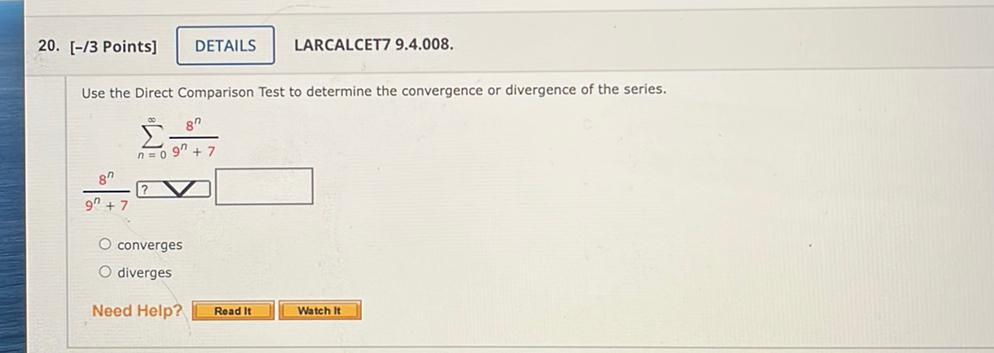Math
Limits and Continuity
Use the Direct Comparison Test to determine the convergence or divergence of the series. ∞ Σ 8^n/(9^n) +7 n=0 a)converges b)diverges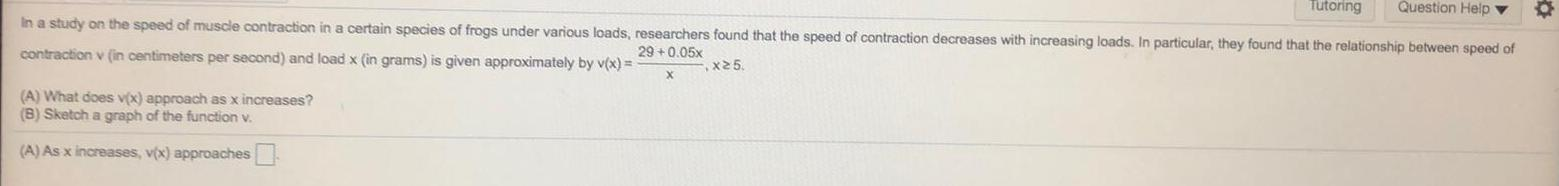Math
Limits and Continuity
In a study on the speed of muscle contraction in a certain species of frogs under various loads, researchers found that the speed of contraction decreases with increasing loads. In particular, they found that the relationship between speed of contraction v (in centimeters per second) and load x (in grams) is given approximately by v(x) = 29+0.05x/x, x≥5 (A) What does v(x) approach as x increases? (B) Sketch a graph of the function v. (A) As x increases, v(x) approaches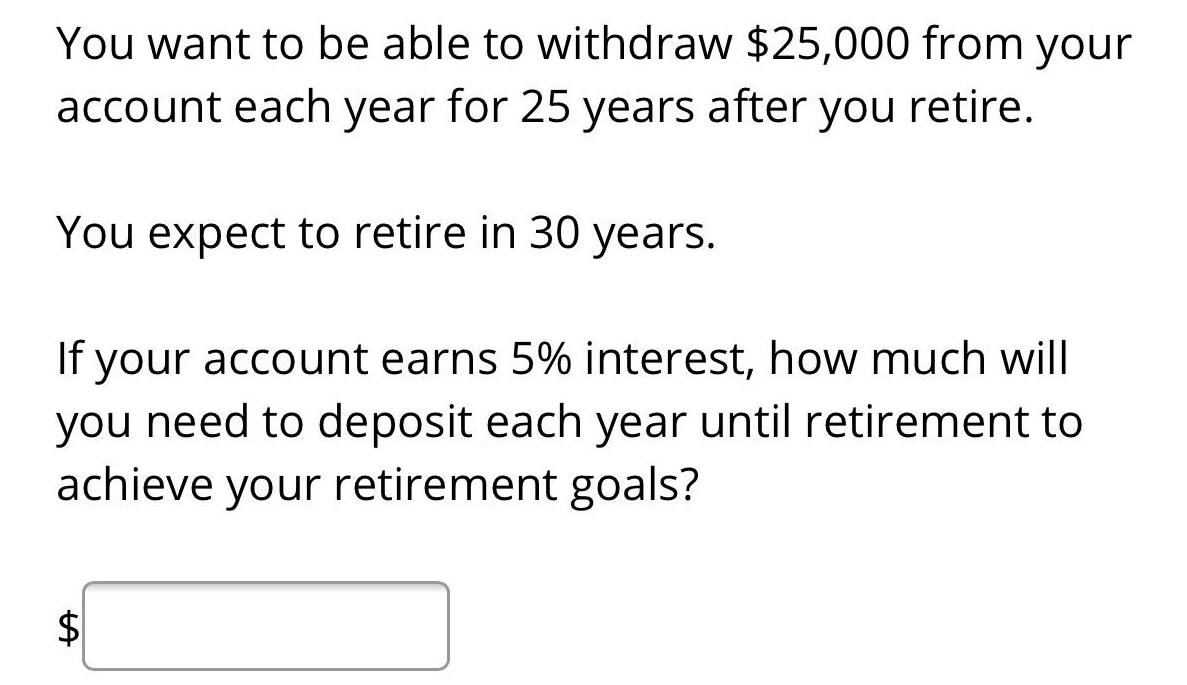Math
Limits and Continuity
You want to be able to withdraw \$25,000 from your account each year for 25 years after you retire. You expect to retire in 30 years. If your account earns 5% interest, how much will you need to deposit each year until retirement to achieve your retirement goals?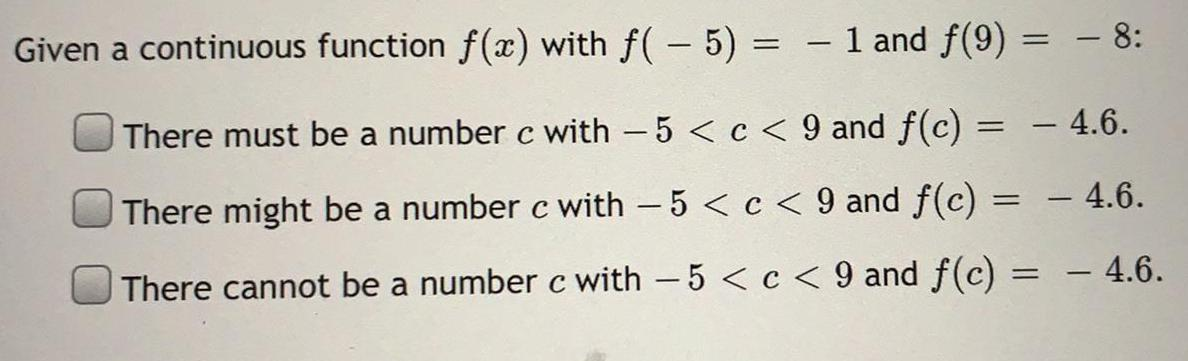Math
Limits and Continuity
Given a continuous function f(x) with f(-5) = - 1 and f(9) = - 8: There must be a number c with − 5 < c < 9 and f(c) = - 4.6. There might be a number c with - 5 < c < 9 and f(c) = - 4.6. There cannot be a number c with -5 < c < 9 and f(c) = - 4.6.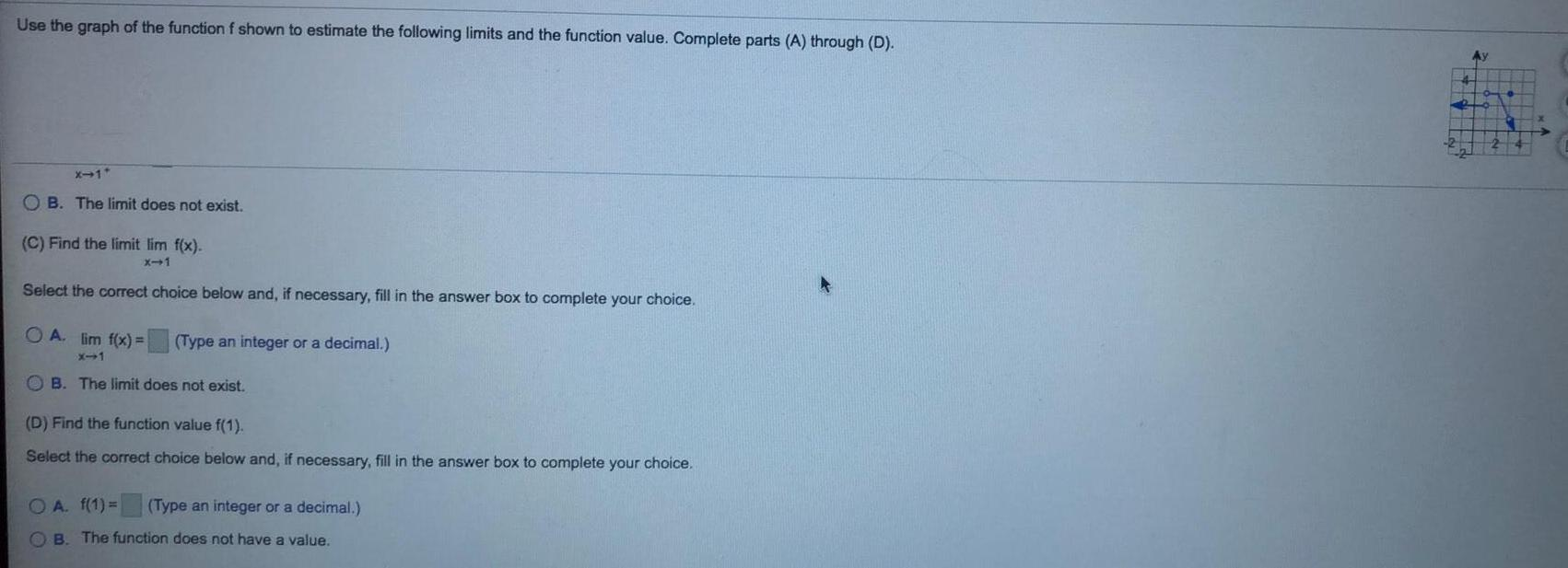Math
Limits and Continuity
Use the graph of the function f shown to estimate the following limits and the function value. Complete parts (A) through (D). (C) Find the limit lim x⇒1 f(x). Select the correct choice below and, if necessary, fill in the answer box to complete your choice. A. lim x⇒1 f(x) B. The limit does not exist. (D) Find the function value f(1). A. f(1) = B. The function does not have a value.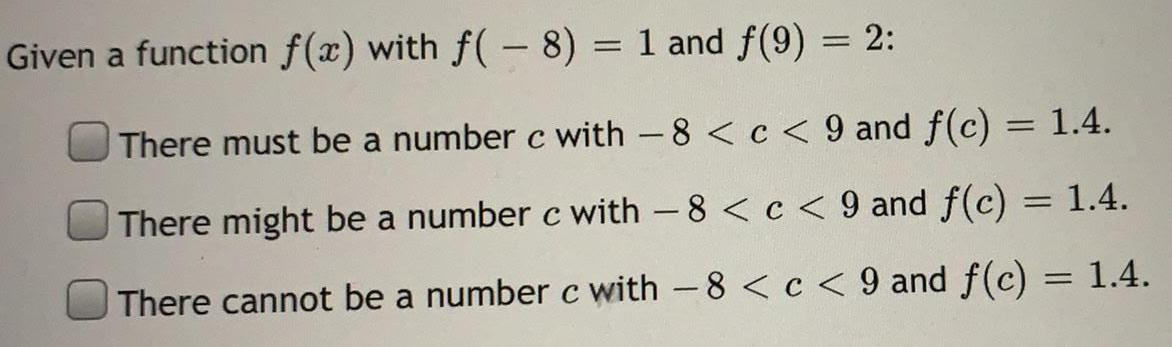Math
Limits and Continuity
Given a function f(x) with f(− 8) = 1 and f(9) ,m= 2: There must be a number c with -8 < c < 9 and f(c) = 1.4. There might be a number c with 8 < c < 9 and f(c) = 1.4. There cannot be a number c with -8 < c < 9 and f(c) = 1.4.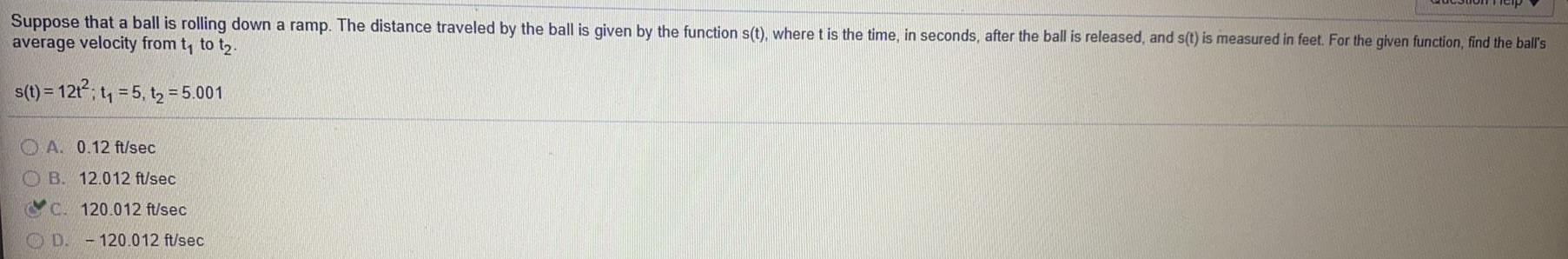Math
Limits and Continuity
Suppose that a ball is rolling down a ramp. The distance traveled by the ball is given by the function s(t), where t is the time, in seconds, after the ball is released, and s(t) is measured in feet. For the given function, find the ball's average velocity from t₁ to t₂. s(t) = 12t²; t₁ = 5, t₂ = 5.001 A. 0.12 ft/sec B. 12.012 ft/sec C. 120.012 ft/sec D. -120.012 ft/sec)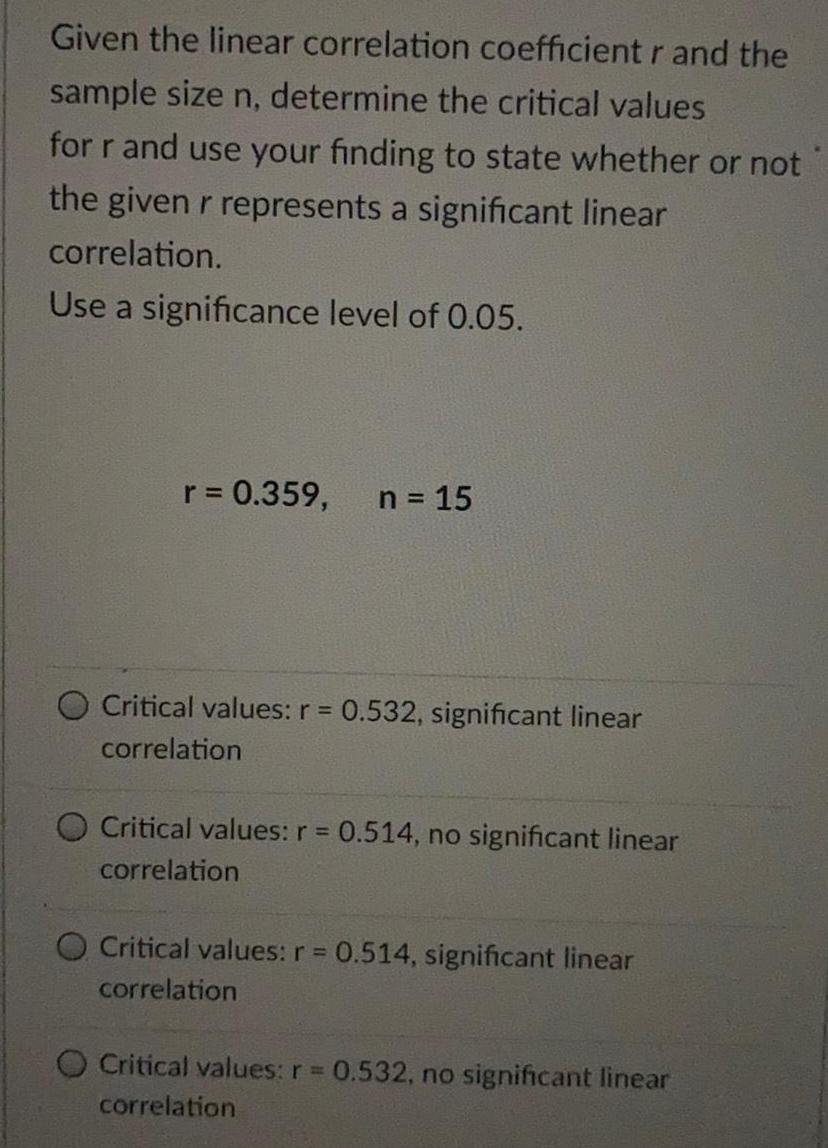Math
Limits and Continuity
Given the linear correlation coefficient r and the sample size n, determine the critical values for r and use your finding to state whether or not the given r represents a significant linear correlation. Use a significance level of 0.05. r = 0.359, n = 15 Critical values: r = 0.532, significant linear correlation Critical values: r = 0.514, no significant linear correlation Critical values: r = 0.514, significant linear correlation Critical values: r = 0.532, no significant linear correlation